# The Origami Polygon Cutting Theorem

### By Eric Biunno

#### Computational Geometry 308-507A

McGill University
Fall 2003
Introduction
Some Theory
Algorithm
Proof of Algorithm
Example Applet

## Introduction

There are many books, websites and papers devoted to origami, each one with its own definition of the word. The Japanese origin of the word simply means "to fold paper". The art of folding paper has been around since at least the sixth century, brought to Japan by Buddhist Monks . When discussing origami, we usually picture elaborate and beutiful paper animals. But we also realize that this art is closely related to mathematics or geometry. This scientific aspect, however, was not extensively studied until about 1980, when Origami mathematics emerged as a field .

There are a multitude of ways to fold paper and different techniques can be used to achieve certain sculptures. In the study of origami mathematics, one would like to relate these notions of 'paper' and 'folds' to mathematical concepts. We can then use existing geometrical theorems to discover new theorems that relate to origami. The theory and algorithm presented in "Folding and Cutting Paper" by Erik D. Demaine, Martin L. Demaine and Anna Lubiw in 1998  will be discussed here.

TOP

## The Origami Polygon Cutting Theorem

The origami polygon cutting theorem tries to solve the fold-and-cut problem. The fold and cut problem can be stated as follows: We are given an initial shape drawn on a piece of paper. Let's fold the paper down to some other flat shape. Next, let's cut our folded paper along one straight line. Now, unfold the pieces of paper. Can we do this so that the boundary of some of our new pieces of paper outline the drawn shape we initialy had? Can we do this for all drawn shapes? We can also look at the problem like this: Given a piece of paper in any shape, can we fold it down flat so that all of the shape's edges end up along one straight line?
Well, the origami polygon cutting theorem is as follows: Given any collection of straight edges, there exists a flat folding and a line in that folding such that cutting along it results in the desired pattern of cuts. .
Let me introduce some preliminary terminology so that we can better understand this theorem.

A plane graph is a planar graph where every edge is straight and has positive length, and every pair of edges intersects only at a shared vertex. Infinite lines, half-infinite lines, and line segments are represented by edges with zero, one, or two actual vertices respectively.

A crease pattern is simply a plane graph (figure 1). This is the collection of lines and segments which will show us where to fold our piece of paper in order to get the shape we want.

We must also know how to fold our paper, or more precisely, which direction we should fold the paper at every edge of our crease pattern. We say an edge of the crease pattern is a mountain edge if it must fold an angle Pi relative to the top side of the paper (fold away from you) and it is a valley edge if it must fold an angle -Pi relative to the top side of the paper (fold towards you).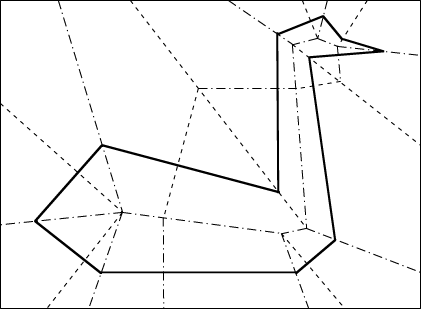Figure 1: A Crease Pattern For A Simple Polygon. Mountain edges are dash-dotted, valley edges are dashed

An origami or folding of a crease pattern is a continuous function from R2 to R3 with the following properties:
1. The function must map every face of the crease pattern to a congruent copy in three dimensions. We can't stretch the paper, if we allowed this, it would tear.
2. The folding must not induce any crossings. When folding, we can't have the paper go through itself. How do we do this?
• Allow faces to be an infinitesimal distance apart.
• Enforce that the folding be a one-to-one function.

3. Note that a folding presents us with the final folded state, not the process of how to get to the folded state.

A flat origami is a folding whose image lies on a single plane. The final state of the folded paper is flatened.

A cut is just a line. We want to remove this line from our plane graph somehow. There are two ways of doing this, each defines a type of cut:
• Mathematical cut: Applying a cut c to some object O is to remove all the points along c from O (O \ c). Cutting an open set results in the resulting pieces also being open sets. This is similar to cutting something with a laser. We are actually burning the points on the cut line. After this cut is made, those points are gone.
• Scissor cut: A scissor cuts without actually removing the line it cuts accross, the points of the cut graph will be on one of the resulting pieces. The effects of this are not different from a mathematical cut in most cases. The difference arizes when a fold line and a cut line coincide, the points on the fold are not removed with a scissor cut (see figure 2).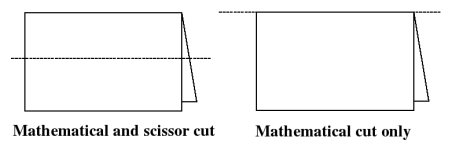Figure 2: Mathematical cuts can cut along the fold line, scissor cuts cannot.

So, we can now see what we want to achieve:
Given a plane graph --which we call the cut graph-- drawn on a piece of paper construct a crease pattern that once folded aligns all the edges on to a single line and nothing else is on that line. This line will be our cut line, and after performing the cut and unfolding our pieces, we will have seperated the regions defined in our cut graph.

TOP

## Algorithm for Solving Fold-and-Cut Problem

Here is a description of the algorithm presented in . Solving the fold-and-cut problem can be split up into two steps:
1. Find a crease pattern.
2. Assign mountain/valley fold directions to each edge of the crease pattern.

### Finding a crease pattern

Straight Skeleton
A natural way to get all the edges of a polygon to line up onto a single line is to fold along the bisector of their extensions. A generalization of this idea to arbitrary cut graphs is the straight skeleton (figure 3). The straight skeleton structure is defined to be the trajectories of the vertices as we shrink the faces of the cut graph. Shrinking consists of continuously insetting each vertex towards the interior of the face, so that at any particular time:
• Every shrunken edge is parallel to the original;
• The perpendicular distance between the shrunken and original boundary edges is the same for all boundary edges.
• A face may split into multiple pieces, in which case we recursively shrink each piece.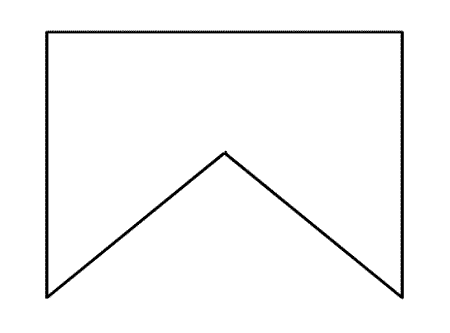Figure 3: Finding the Straight Skeleton of a Polygon.

There are a few algorithms for finding the straight skeleton of a given plane graph: Felkel & Obdrzálek , Aichholzer & Aurenhammer . A very good explanation of the straight skeleton along with an interactive applet can be found at David Bélanger's Designing Roofs of Buildings. We are not too concerned with the straight skeleton algorithm although the running time and the resulting crease pattern are dependent on it. The fatsest algorithm to date runs in O(n17/(11+E)). We are instead more interested with the properties of the straight skeleton itself.

Properties of the Straight Skeleton
Let us first note that the straight skeleton is a plane graph. Here are some other properties:
• If n is the number of vertices in the given cut graph, then the straight skeleton has O(n) vertices, edges, and faces.
• Every edge in the cut graph is contained in exactly one skeleton face, and every skeleton face contains exactly one edge in the cut graph.
• Finally, a skeleton edge incident to a vertex of the cut graph bisects the angle of that vertex.
We should also note that the typical, non-degenerate, vertex of a straight skeleton has degree 3. This will give us a final folding which will not lie on a plane since we need even degree vertices for a graph to be flat foldable. So we must somehow add more fold edges...

Perpendiculars
The main idea here is to add a fold perpendicular to an edge of the given cut graph. This would maintain the property that the edges of the cut graph line up. Let us define what a perpendicular is and how to obtain them. The perpendicular associated with any point p in the plane consists of a collection of line segments, rays, and lines called perpendicular edges, each associated with a straight skeleton face.
A recursive definition of perpendicular edges is as follows: For each closed skeleton face that the point p is in, create a line segment going through p and perpendicular to (the line extending) the edge of the cut graph associated with the skeleton face. The line segment has endpoints at the boundaries of the face and is called a perpendicular edge associated with that face. Then the perpendicular associated with p contains both this line segment and the perpendiculars associated with the endpoints of this line segment. So, every time a pependicular hits any othe edge it bounces, or the segment is reflected by that edge and keeps going until it hits another edge.

We can seperate perpendiculars into two categories, real perpendiculars and imaginary perpendiculars. A real perpendicular is a perpendicular that is incident to a vertex of the straight skeleton of the cut graph. Imaginary perpendiculars are simply all other perpendiculars. Together, all of the real perpendicular edges form the perpendicular graph (see figure 4).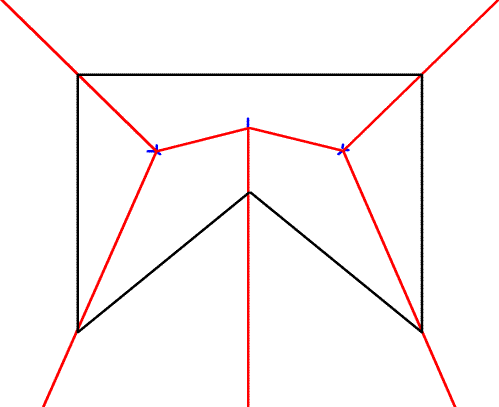Figure 4: Finding the Perpendicular Graph of a Polygon.

There are two noteworthy properties of perpendiculars:
• There are O(n) real perpendiculars.
• The perpendicular edge associated with a skeleton face is perpendicular to the edge of the cut graph associated with that face; in particular, all such perpendicular edges are parallel.

### Mountain-Valley Assignment

The next step of the algorithm involves labeling the edges of the crease pattern as mountain or valley edges. The assignment is performed according to whether the faces of the cut graph lie on one side of the cut line or the other. For faces of the given plane graph that are above the cut line:
• Any skeleton edge incident to a convex vertex of the face is folded as a mountain and any edge incident to a reflex vertex is folded as a valley.
• Perpendiculars change assignment every time they bounce. They initially start out as a valley if incident to a convex vertex, and a mountain if not.
For faces below the cut line, the edges have the opposite assignment. Examples of assignments can be seen in figure 1 and figure 5.
Now we can fold our original cut graph and cut along the cut line (the edges of the cut graph), to get the desired result. This is the algorithmic aproach used in  to solve the fold-and-cut problem for a given cut graph.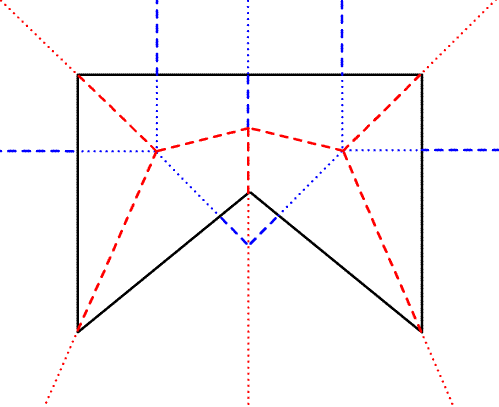Figure 5: Assigning Mointain (dashed) or Valley (dotted) Folds To Edges.

TOP

## Proof of Algorithm's Correctness (Outline)

Corridors
In order to prove the above algorithm let us examine two other structures related to perpendiculars, corridors. Corridors are simply the faces of the perpendicular graph. Unlike in usual plane graphs, a corridor never consists of a bounded simply connected region. We can classify corridors in two ways: by topology and by wall count.

Two classifications of corridors:
1. By Topology:
• linear: corridor's interior is homeomorphic to an infinite band.
• circular: interior is homeomorphic to an annulus.
2. By Wall Count:
• A wall is one of the perpendiculars that bound the corridor.
• A corridor can have one or two walls
There is a nice lemma which says: Every corridor D is either linear or circular but not both, and has either one or two walls. Furthermore, D has constant width. So from this lemma, there are only four types of corridors possible, which are shown in figure 6.Figure 6: The Four Types of Corridors

Accordions
The other structure we wish to examine is called an accordion (figure 7). Consider a corridor, the creases intersecting it, and the mountain-valley assignment for those creases. An accordion is a folded state of this.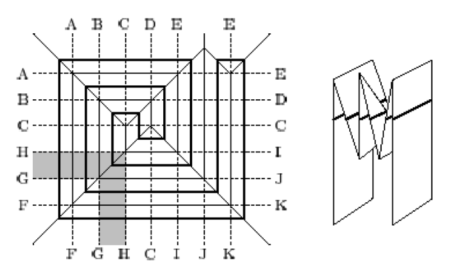Figure 7: Accordion: Folding A Corridor

Let us prove the algorithm described above solves the fold-and-cut problem for crease patterns which have no circular corridors. To do this we must first prove that an accordion exists and lines up all edges of the cut graph contained in its corridor.
If creases completely cross the corridor, then a folded state exists:
• Perpendiculars are the corridor walls, we do not fold them.
• Straight skeleton edges are not a problem since they are always in a 2-wall corridor
• Edges of the given cut graph may be a problem. But let's consider an imaginary perpendicular incident to a vertex of the cut edge. Then this edge is completely contained in this new subdivided corridor.
Lining up of edges within an Accordion:
• The creases in a subdivided corridor alternate between mountain and valley, thus the accordion does not self-intersect.
• A fold at a skeleton edge lines up the two cut edges bisected by the skeleton edge.
• Because each wall of the (subdivided) corridor is perpendicular to the cut edges, folding at the skeleton edge causes the two incident perpendicular edges in the wall to fold to a common line. This is also the case when we fold at a cut edge.
Next, we use the following lemma to combine all the accordians we have and get the final folding. Lemma: Two adjacent corridors fold into accordions that match up at their common side.
Finally we have to show that this folding can be folded flat (folded down to a single plane). We can do this by modeling accordians by a tree:
Folding this collection of accordions flat onto one plane reduces to folding a tree (figure 8).
This tree corresponds to the xy projection of the folded model:
• Each edge corresponds to an accordion.
• Each vertex corresponds to a side of an accordion.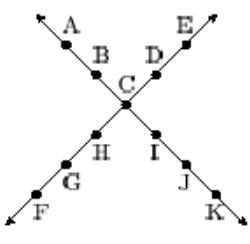Figure 8: Folding of A Tree.

TOP

## Sample Applet

This Java Applet attempts to create the appropriate crease pattern for a given simple polygon. Once you have finished drawing a simple polygon, you can see the shape's straight skeleton and perpendiculars by selecting the appropriate buttons. It also can show you which way to fold your paper (i.e. Mountain-Valey assignment of edges). This applet will not compute the minimum set of perpendiculars, but as noted before extra perpendiculars don't matter.

To start the applet make sure you have the latest Java Runtime Environment installed, and click the button below, it will open in a pop-up window. You can also save a screenshot and print out the shape on to paper, and try it out.
Any simple polygon can be drawn, but computation of the straight skeleton is only guaranteed for convex polygons in this implementation. It may compute the correct straight skeleton for non-convex polygons. The perpendiculars depend on the straight skeleton and are correct, assuming the straight skeleton is correct. Mountain-Valley assignments are also correct for convex polygons.
This applet is inspired by, and shares some code with David Bélanger's Applet .

TOP